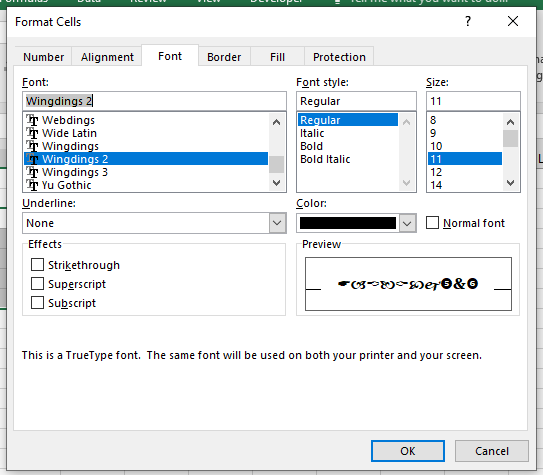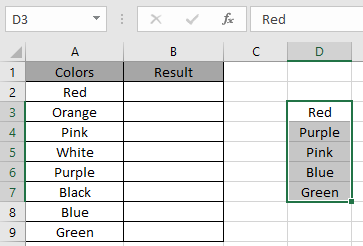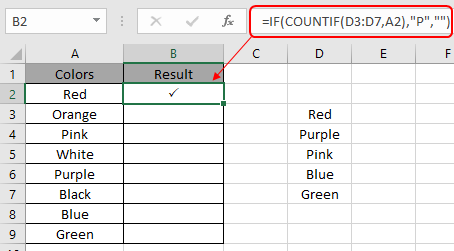# Validate Input With Check Marks in Excel

In this article, we will learn about how to use the IF function to put check marks in for valid inputs in Excel.

The check mark option is enabled in the format cell option. Use the Ctrl + 1 shortcut on the selected cell and Format Cells dialog box appears in front. Select the font option and then select Wingdings 2 under Font and customize the Font style and size as required. Wingdings 2 operates capital P as a check mark in Excel.The IF function tests the condition and returns value whether it's True or False.
The COUNTIF function of excel just counts the number of cells with a specific condition in a given range.

Syntax for putting Check Mark in Excel

= IF ( true_false_statement, "P" , "" )

true_false_statement:It is any statement that can be used as true false values.

Example:

Here we have some color names in column A. in range D3:D7, we have valid color names. In B column we need to put check marks if A column contains colors from range D3:D7.Here we need to use the formula to get the check mark symbol wherever required.

We will use the formula to get the check mark.

= IF ( COUNTIF ( D3:D7 , A2 ), "P" , "" )

Explanation for the formula:

The COUNTIF function will return a value greater than 0 if the value is found at least once, else returns 0. So the COUNTIF function works fine logic test for the IF function.
The IF function returns capital P if the value is 1. So whenever the value is valid, excel will put a check mark in adjacent cell.  Else it returns an empty string if the value is 0.Here the arguments to the function is given as cell reference. Freeze the D3:D7 referenced cell and Copy the formula to the remaining cells using the Ctrl + D shortcut or dragging down from right bottom of the resultant cell in excel.As you can see the check marks are put wherever required.

Notes:

1. The function returns an error if a non numeric value is used without a quote sign ("value").
2. The function returns the check mark using the IF function with COUNTIF function.

Hope it was explanatory. Now you know how to Validate input values with check mark in Excel. Explore more articles on the Excel IF function here. Please feel free to state your query or feedback for the above article.

Related Articles

IF function with wildcards

How to Use Nested IF Function in Excel

Using If Function to Compare Dates of Two Cells

How to use the IF Function in excel

How to use the COUNTIF function in Excel 2016

Popular Articles

3 SUMIF with Or Formulas

How to Use SUMIFS Function in Excel

SUMIFS using AND-OR logic

SUMIF with non-blank cells

SUMIFS with dates in Excel

Edit a dropdown list

If with conditional formatting

If with wildcards

Vlookup by date

Terms and Conditions of use

The applications/code on this site are distributed as is and without warranties or liability. In no event shall the owner of the copyrights, or the authors of the applications/code be liable for any loss of profit, any problems or any damage resulting from the use or evaluation of the applications/code.